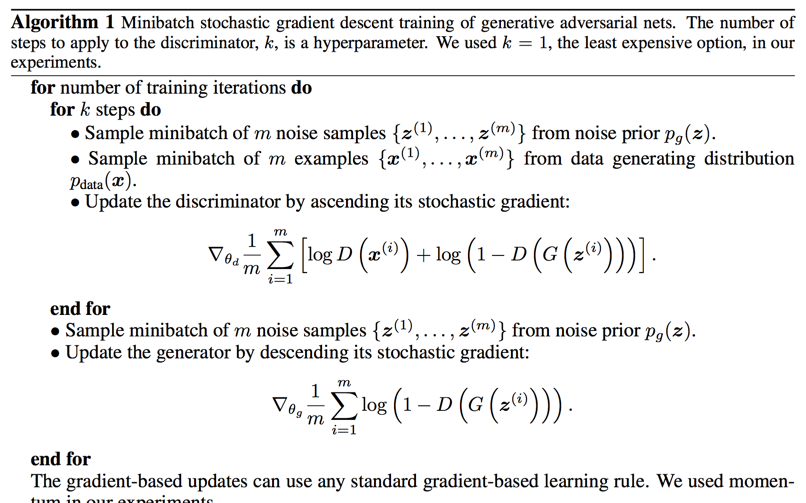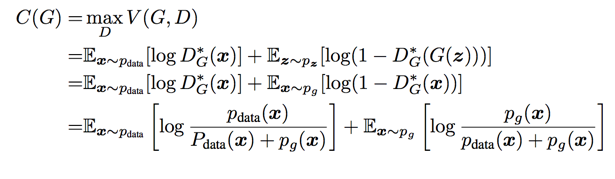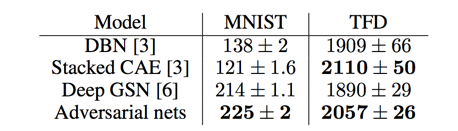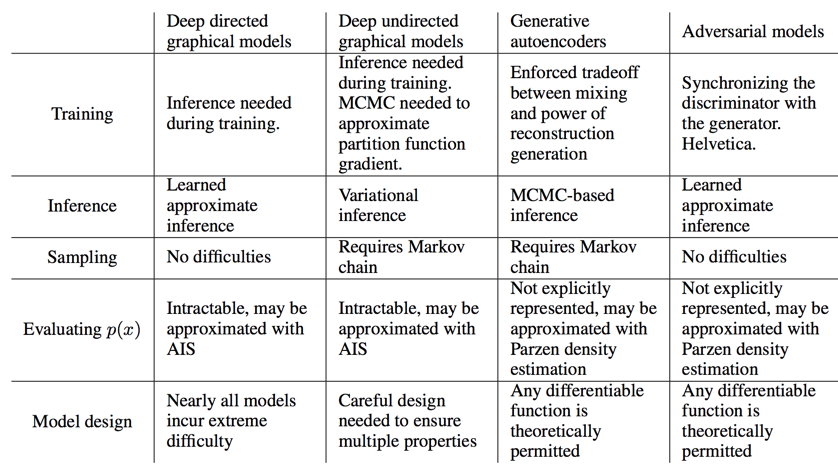0%

## 总结

• 生成对抗网络由生成器G和分类器D组成，生成器G接受噪音生成样本，D负责区分这些样本是否是由D生成出来的
• D和G共同优化目标函数$\underset {G} {min} \underset {D} {max} V(D,G) = E_{x \sim p_{data}(x)}[log(D(x))] + E_{x \sim P_{g_z(z)}}[log(1 - D(g(z)))]$ 一个是让值尽量大，一个是让值尽量小，即所谓“对抗”
• 该目标函数会在$p_g = p_{data}$处收敛到最优，即生成分布与训练数据分布完全一致函数值为$-log4$
• 关于收敛性的证明：一个凸函数集合的上界的次梯度（可导的时候等于梯度）完全包含最后函数的梯度，所以在求导做梯度下降的时候整体仍然按照凸函数的性质收敛
• 使用Gaussian Parzen window(youtube视频)方法对模型进行评估, Parzen Window 其实是用已有数据到待测数据点的“距离”平均值来表示待测点出现在已有数据表示的分布上的概率（或者说在已有数据拟合出的概率密度函数上的值）
• 通用概率密度函数$P(x) = \frac {1} {n}\sum_{i = 1}^{n}\frac {1} {h^d}K(\frac {x - x_i} {h})$
• Parzen window中的核函数可以有多种，使用Gaussian核的就叫做Gaussian Parzen window$P(x) = \frac {1} {n} \sum^n_{i = 1}\frac {1} {h\sqrt {2\pi}^d}exp(-\frac 1 2(\frac {x - x_i} {h})^2 )$
• 算出概率之后再过一遍$score = -log(1 - P(x))$(猜测)
• 优点
• 不使用马尔科夫链
• 训练模型的时候不需要inference
• 可拟合sharp的分布
• 训练样本并不直接作用于G的训练，保证参数独立
• 可使用多种模型拟合G和D
• 缺点
• G和D需要同步训练（两次D一次G）
• 没有$p_g(z)$分布的显示表示

## Abstract

propose a new framework for estimating generative models via adversarial process

simultaneously train two models:

• a generative model G
• a discriminative model D

a unique solution exists, with G recovering the train data distribution and D equal to $\frac 1 2$ everywhere

## Introduction

a discriminative model that learns to determine whether a sample is from the model distribution or the data distribution. The generative model can be thought of as analogous to a team of counterfeiters

## Relate Work

• restricted Boltzmann machines(RBM)
• deep Boltzmann machines(DBMs)
• deep belief networks(DBNs)
• score matching
• noise-contrastive estimation
• generative stochastic network

## Adversarial nets

G is a differentiable function to output a generate data by $G(z;\theta_g)$

D is a multilayer perce ptron $D(x;\theta_d)$ to output a single scalar that represent whether a data is fabricated by G

D and G play the following two-player minimax game with value function $V(G, D)$

we alternate between k steps of optimizing D and one step of optimizing G

Early in learning, when G is poor $log(1 - D(G(z)))$ saturates. we use $\underset {G} {max} logD(G(z))$ instead. It’s provides much stronger gradients early in learning

## Theoretical Results### Global Optimality of $p_g = p_{data}$the global minimum of the virtual training criterion $C(G)$ is achieved if and only if $p_g = p_{data}$. At that point, $C(G)$ achieves the value - $log4$

Since the Jensen–Shannon divergence between two distributions is always non-negative and zero only when they are equal

### Convergence of Algorithm 1

If G and D have enough capacity, and at each step of Algorithm 1, the discriminator
is allowed to reach its optimum given G, and $p_g$ is updated so as to improve the criterion

proof:
The subderivatives of a supremum of convex functions include the derivative of the function at the point where the maximum is attained

## Experiments

database:

• MNIST
• TFD
• CIFAR-10

activation:
generator use mixture of rectifier linear and sidmod as activations; discriminator use maxout activation

We estimate probability of the test set data under $p_g$ by fitting a Gaussian Parzen window to the samples generated with G and reporting the log-likelihood under this distribution## Advantages and disadvantages

disadvantages:

• there is no explicit representation of $p_g(x)$
• and that D must be synchronized well with G during training (in particular, G must not be trained too much without updating D, in order to avoid “the Helvetica scenario” in which G collapses too many values of z to the same value of x to have enough diversity to model $p_{data}$)

advantages:

• Markov chains are never needed
• no inference is needed during learning
• a wide variety of functions can be incorporated into the model
• generator network not being updated directly with data examples, but only with gradients flowing through the discriminator
• they can represent very sharp, even degenerate distributions## Conclusions and future work

extensions in future:

• A conditional generative model $p(x | c)$ can be obtained by adding c as input to both G and D
• Learned approximate inference can be performed by training an auxiliary network to predict z given x
• Semi-supervised learning